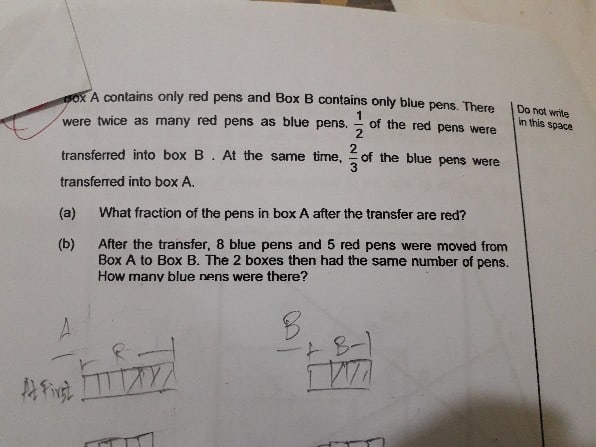QuestionHow to solve

It would be easier if the pens are considered as 1 unit, ignoring the colors.

Initially,

Box A = 6U  => Red

Box B = 3U  => Blue

First transfer

Box A = 3U (R) + 2U (B)

Box B  = 1U(B) + 3U (R)

Second transfer  8 blue pens and 5 red pens are transferred from A  to B.

13 pens are transferred from A to B.

Box A has 5U

Box B has 4U

Since they both will be equal after 13 pens are transferred from A to B,

5U = 4U + 26

1U = 26.

Total blue pens = 3U = 26 x 3 = 78

0 Replies 0 Likes